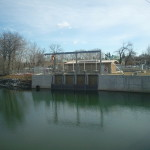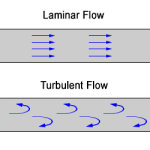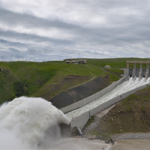## Fluid DynamicsFluid dynamics refers to the study of fluid in motion. It includes fluid moving through pipes, measurements with venturis and orifices, and other motion-related topics such as lift and drag, and pumps. Reference The field of fluid dynamics is a subcategory of fluid mechanics. It’s sibling is fluid statics. In practice, the field of fluid […]

## How to calculate Turbulent FlowTurbulent flow is rough and choppy. Turbulent flow is the opposite of laminar flow, which is straight line flow. In pipelines, laminar flow occurs when Reynolds number is greater than 4,000. If a stream of dye is inserted into the flow and it disperses and mixes, the flow is turbulent. When the flow is turbulent, […]

## How to determine Laminar FlowLaminar flow is smooth flow in a straight line. Laminar is the opposite of turbulent flow. In pipelines, laminar flow occurs when Reynolds number is less than 2,100. If a stream of dye is inserted into the flow and it continues in a straight, unbroken line, the flow is laminar. Flow Types The opposite of […]

## How to Calculate Reynold’s NumberThe Reynolds number of a fluid is a dimensionless constant which allows you to determine whether the flow of a fluid is laminar or turbulent. It represents the ratio of inertial forces to viscous forces in the fluid. The equation is: Where: De = Hydraulic Diameter (ft or m) v = velocity of the fluid […]